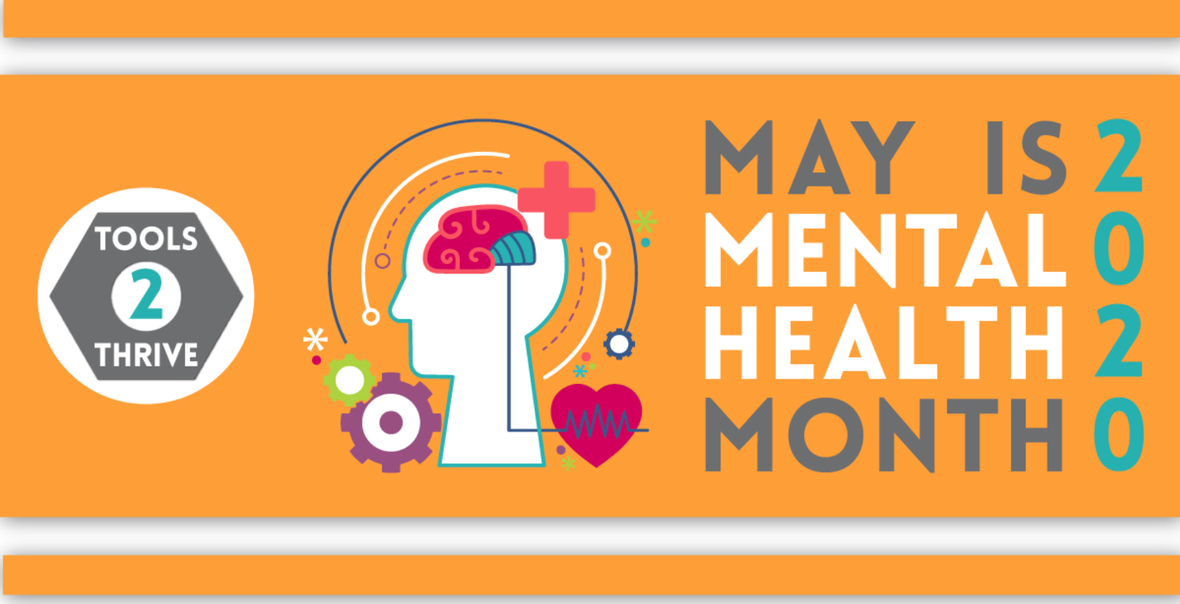## Resolution to Lifestyle

 /* styles */ No Matter The Day - It Will Be Music2YourFeet! -Denise Bryant, Founder
 table div table+table+table+table+table+table div table{width:100%;padding:0}table div table+table+table+table+table+table div table img{width:96.23%;padding:0;float:none}table div table+table+table+table+table+table div table td{width:100%;padding:0 1.88% 18px}/* styles *//* styles */

May is Mental Health Month, and it has never been more important than this year.

Just weeks ago, we had no idea that all our worlds were going to be turned upside down by the coronavirus. Or that the associated worry, isolation, loneliness, and anxiety would be something that literally everyone – would experience.

Mental health screening is essential during times of stress. Take a screen. And tell your friends to take a screen, too. It is more important than ever to take care of our mental health.

It is important to look forward and to focus on what we need to do to thrive in uncertain times. There are the tools that will also help us do just that.
May you stay safe and well!

Tools for you to use
----------

## Finding the positive

ChooseBeauty.org - A website on a mission to lift your spirits - giving you strength, comfort, and light when you need it most.

Positive Psychology Center - UPenn’s online hub for positive psychology research and learning at ppc.sas.upenn.edu.

## Healthy Routines

ColorTherapy.app - The Color Therapy app promotes relaxation and mindfulness through social coloring and painting. Available for Apple devices. or Download Creating Healthy Routines handout

ThisIsMyBrave.org - A speaker’s bureau or show whose mission is to bring stories of mental illness and addiction out of the shadows and into the spotlight.

Yale Center for Emotional Intelligence - Yale’s online hub for emotional intelligence research and resources at ei.yale.edu.

## Eliminating Toxic Influences

National Domestic Violence Hotline - Available 24/7, 365 days a year, the National Domestic Violence Hotline provides support and resources for people who are being abused or people that suspect someone they care about is being abused. Services are available in multiple languages. Call 1-800-799-SAFE (7233) or text “LOVEIS” to 22522. Visit thehotline.org for additional information.

## Connecting with and supporting Others

Clubhouse-Intl.org - A website for individuals living with mental illnesses to find Clubhouses—safe environments with opportunities for friendship, employment, housing, education and access to medical and psychiatric services in a single setting.

HappyTheMovement.com - Happy the App allows you to receive support and encouragement from compassionate people. Available for Apple and Android devices.

Inspire.com - A social network for patients and caregivers to connect, share, and learn from each other about medical conditions, treatment, and support.

MakeSureYourFriendsAreOkay.com - Through merchandise and social media, Make Sure Your Friends Are Okay is building a
community of like-minded people who want to help get the world talking.

TheMighty.com - A safe, supportive online community for people facing health challenges and the people who care for them.

YMHProject.org - The Youth Mental Health Project offers The Parent Support Network, a parent-driven, family-focused program that provides parents who are concerned about their children’s mental health with a dependable and caring network, valuable peer support, and access to peer-recommended resources in their local communities.

## Mental HEALTH CONDITIONS

ChoicesInRecovery.com - Support and information for people with Schizophrenia, Schizoaffective, and Bipolar Disorder and their caregivers, including resources for having conversations with treatment professionals.

MoreThanMyDiagnosis.com - Stories and advice from people who either live with mental health issues or care for someone who does.

Here's to your Health in 2020!
Denise

Source: Mental Health National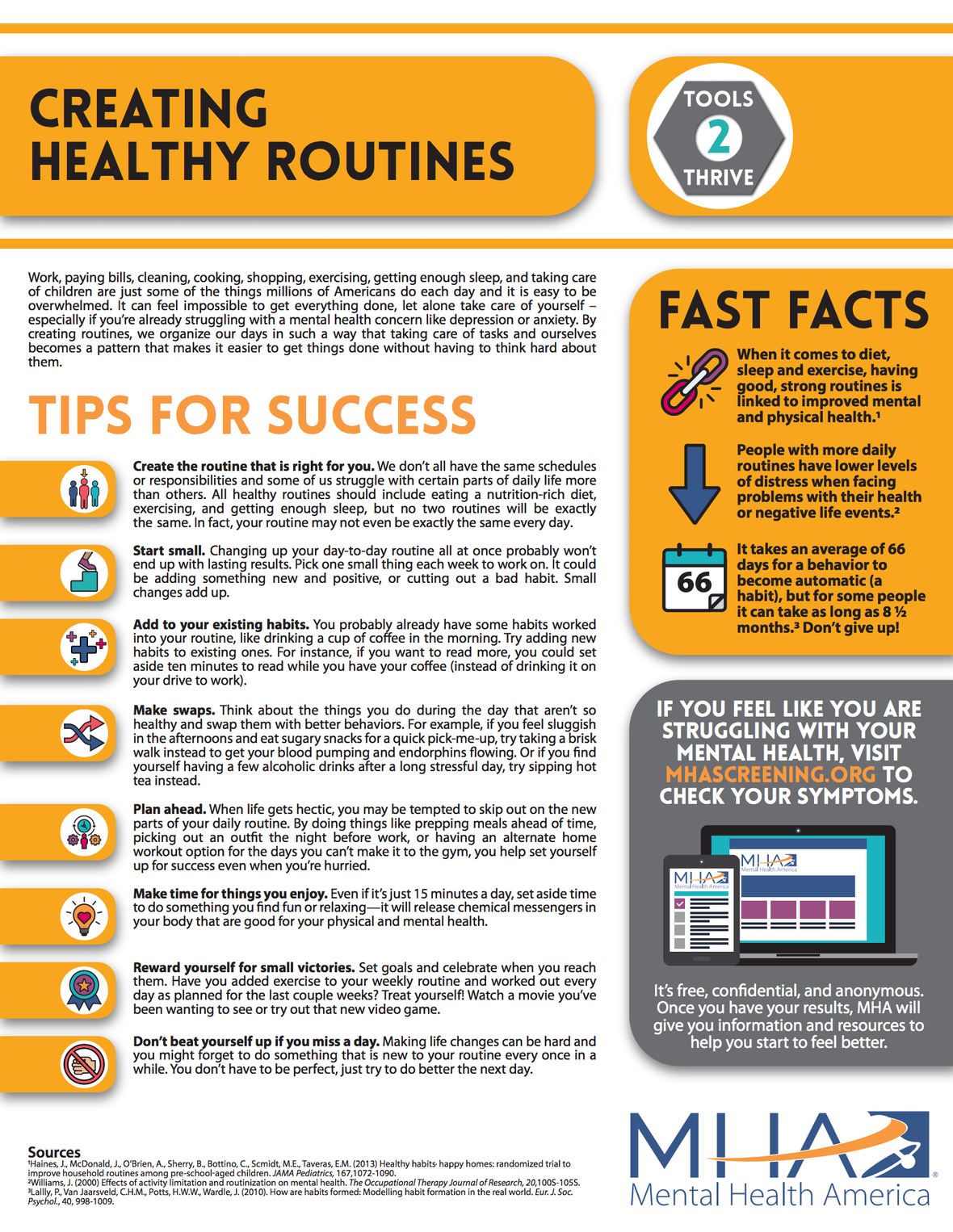table div table+table+table+table+table+table+table+table+table+table div table{width:100%;padding:0}table div table+table+table+table+table+table+table+table+table+table div table img{width:96.23%;padding:0;float:none}table div table+table+table+table+table+table+table+table+table+table div table td{width:100%;padding:0 1.88% 18px}/* styles */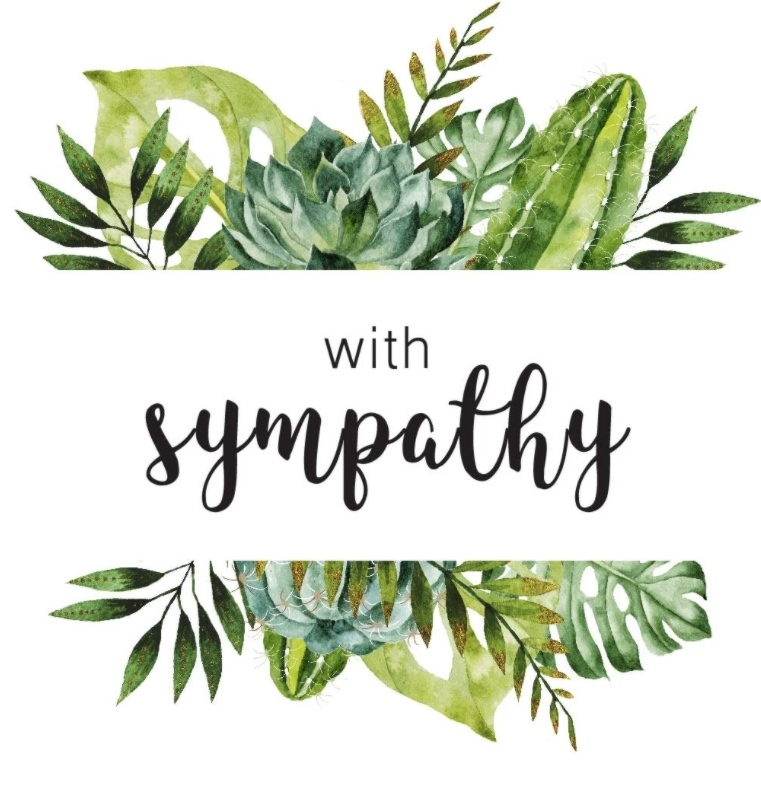/* styles */ Condolences to everyone who has lost a loved one due to the COVID 19. God bless you and your family. God bless everyone who has lost moms, dads, sisters, brothers, family, friends, relatives, cousin's, co-workers, and line dance family. Rest in Heaven, keep praying family. Condolences to the Richardson family, Keith & Michael on the death of your mom, Ms. Bennie Mae Richardson, a long time neighbor and very special family friend, we are going to miss you. Rest In Peace. Condolences to the Chittams Family, on the death of their loved one, Carolyn Chittams, our very good friend, we will miss you, Rest in Heaven. Condolences to The Jones family, on the death of their Mom, Betty Jones May she Rest In Peace , always in our heart.

## Denise's Corner

Special memory of our mom

## Delores Veronica Kidwell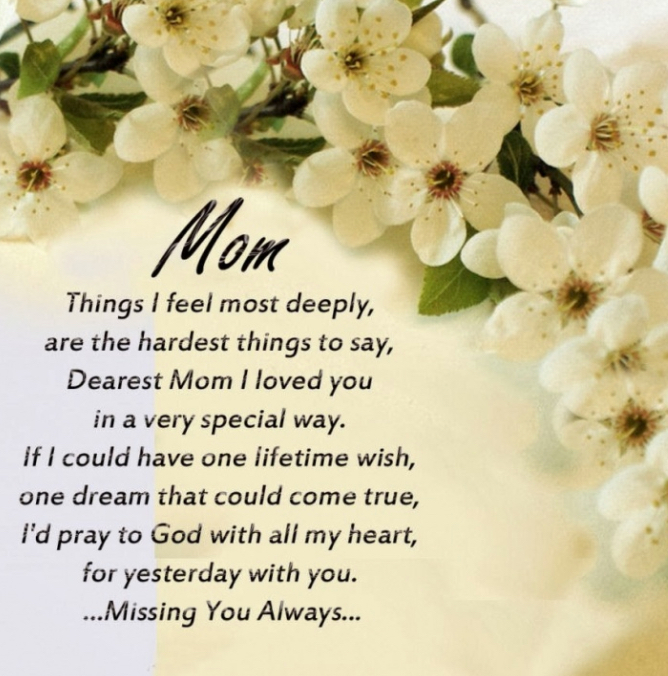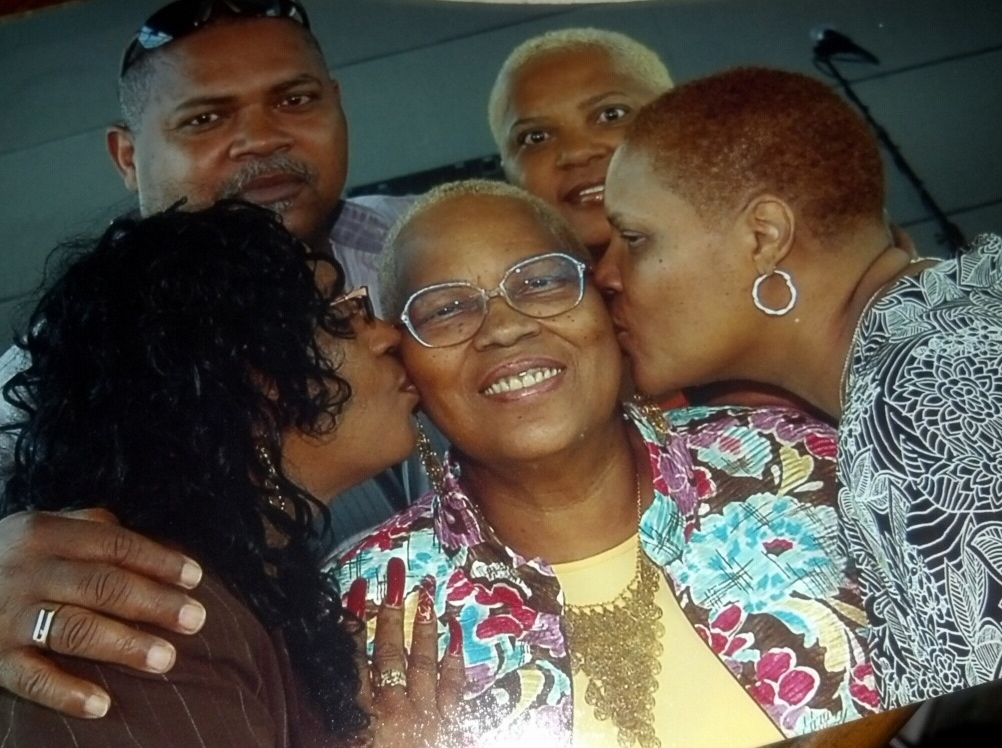/* styles */ It will be 4 years, on May 28, 2016; that you had to leave us, we miss you as if it was yesterday, We know you are in a better place, but the emptiness we feel in our hearts will never be filled, we love you.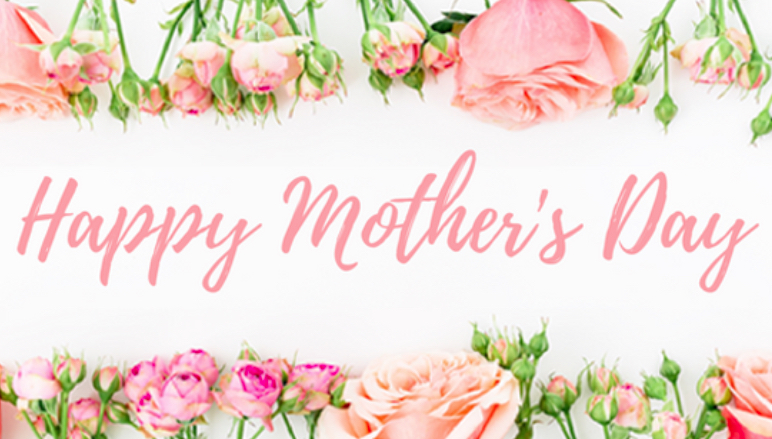table div table+table+table+table+table+table+table+table+table+table+table+table+table+table+table+table+table+table+table div table{width:100%;padding:0}table div table+table+table+table+table+table+table+table+table+table+table+table+table+table+table+table+table+table+table div table img{width:96.23%;padding:0;float:none}table div table+table+table+table+table+table+table+table+table+table+table+table+table+table+table+table+table+table+table div table td{width:100%;padding:0 1.88% 18px}/* styles */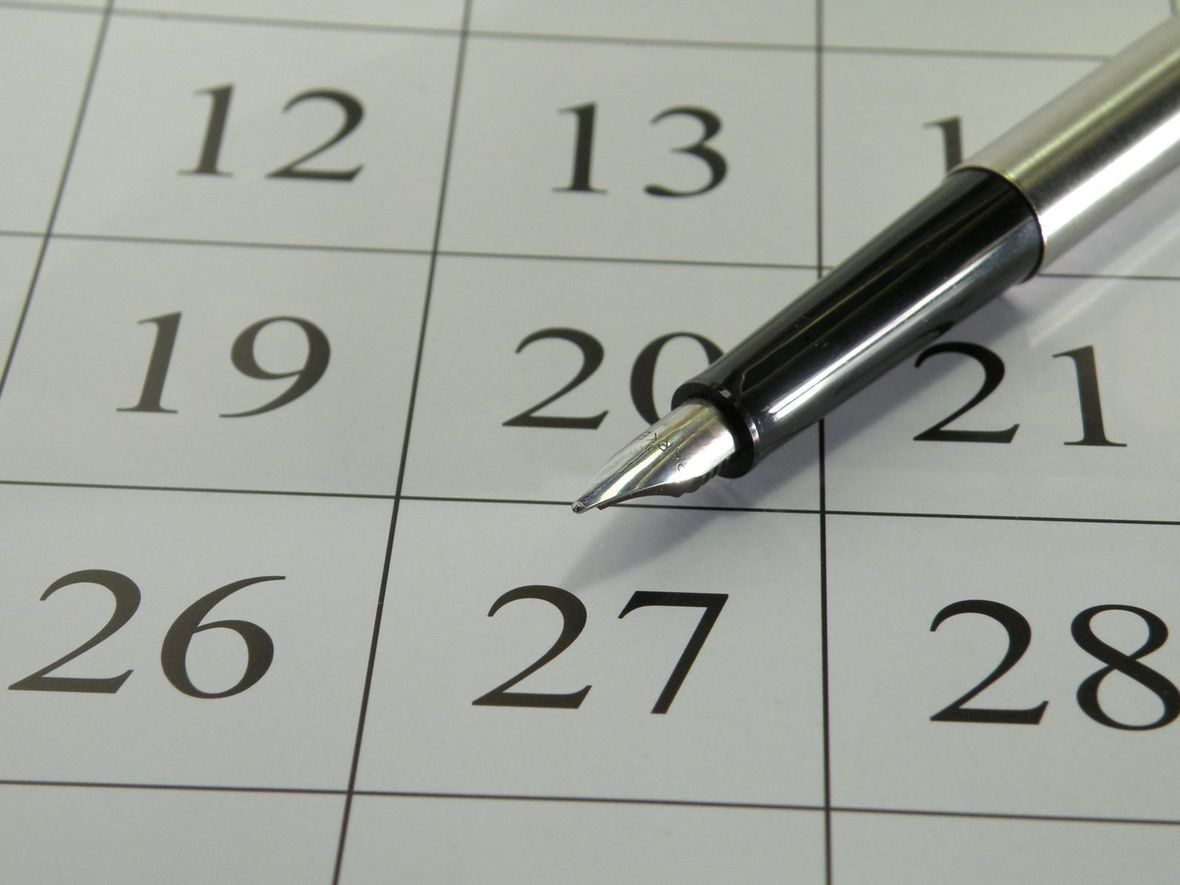# Music2yourfeet 7th Annual Holiday Party

 table div table+table+table+table+table+table+table+table+table+table+table+table+table+table+table+table+table+table+table+table+table div table{width:100%;padding:0}table div table+table+table+table+table+table+table+table+table+table+table+table+table+table+table+table+table+table+table+table+table div table img{width:96.23%;padding:0;float:none}table div table+table+table+table+table+table+table+table+table+table+table+table+table+table+table+table+table+table+table+table+table div table td{width:100%;padding:0 1.88% 18px}/* styles */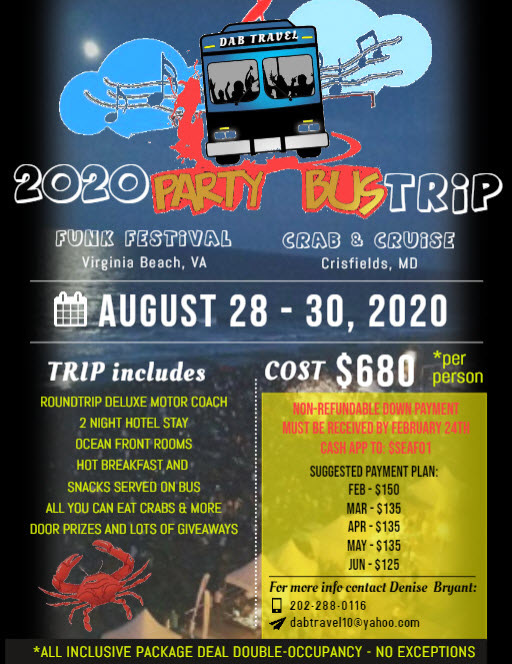# Due to the coronavirus outbreak the 2020 Party Bus Trip is canceled.

 table div table+table+table+table+table+table+table+table+table+table+table+table+table+table+table+table+table+table+table+table+table+table+table div table{width:100%;padding:0}table div table+table+table+table+table+table+table+table+table+table+table+table+table+table+table+table+table+table+table+table+table+table+table div table img{width:96.23%;padding:0;float:none}table div table+table+table+table+table+table+table+table+table+table+table+table+table+table+table+table+table+table+table+table+table+table+table div table td{width:100%;padding:0 1.88% 18px}/* styles */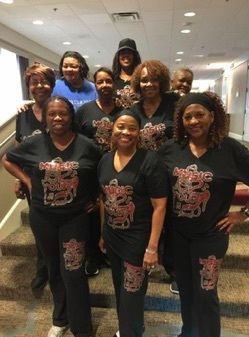Music2YourFeet Workout Suit (2 Piece)

\$40 for set, separate \$20 each,
come in black and gray only, stretch material...size from small to 2x pants and 3X shirt, may use Cash App \$seafo1 to pay, or cash, sorry no paypal and no checks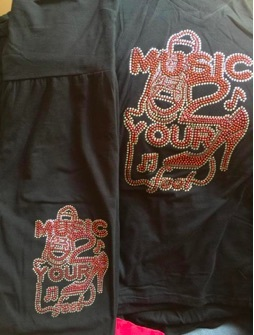Music2YourFeetWorkout Suit (pant and t-shirt)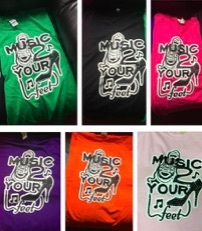Music2YourFeet T-Shirt

\$15 each, may use Cash App \$seafo1 to pay, or cash, sorry no paypal and no checks

Please indicate the T-shirt color when ordering.
Colors available:
Green, Black, Hot Pink, Purple, Orange and Light Pink,

 table div table+table+table+table+table+table+table+table+table+table+table+table+table+table+table+table+table+table+table+table+table+table+table+table+table+table+table+table div table{width:100%;padding:0}table div table+table+table+table+table+table+table+table+table+table+table+table+table+table+table+table+table+table+table+table+table+table+table+table+table+table+table+table div table img{width:96.23%;padding:0;float:none}table div table+table+table+table+table+table+table+table+table+table+table+table+table+table+table+table+table+table+table+table+table+table+table+table+table+table+table+table div table td{width:100%;padding:0 1.88% 18px}/* styles */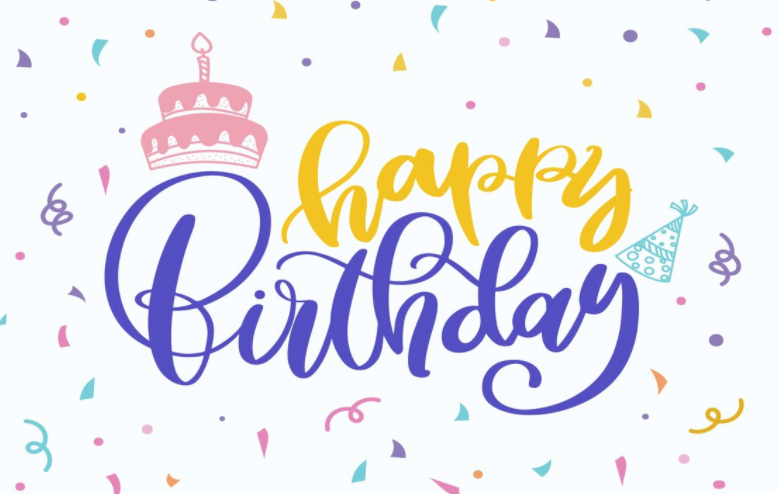## Special Birthday shout outs to my family and friends....

 /* styles */ A very Special Happy Sweet 16th Birthday to Talia Thomas, May God keep you safe in his loving arms, we love you, enjoy your bday. A very Special Happy Birthday in a Heaven to my daughter by another mother, Maryam Thomas, we miss you so much, And wish you were still here with us, continue to Rest In Peace, sweet angel, we love you. A very Special Happy Birthday to my beautiful daughter, Kiana aka ween, aka toy, aka aunt Kiana and younger sister, Vernita, aka, Muff, Ms. V, aka aunt Muff.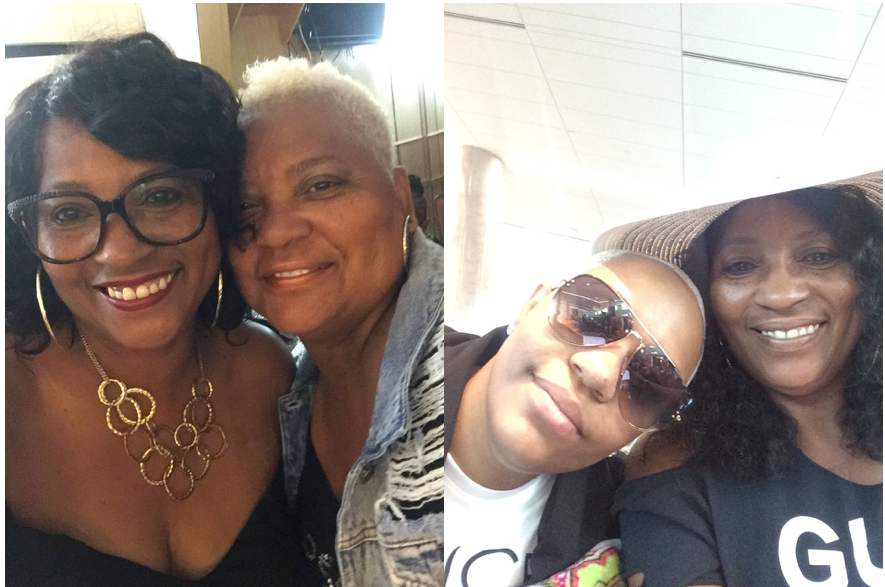Click here to view a special birthday photo gallery of Kiana and Vernita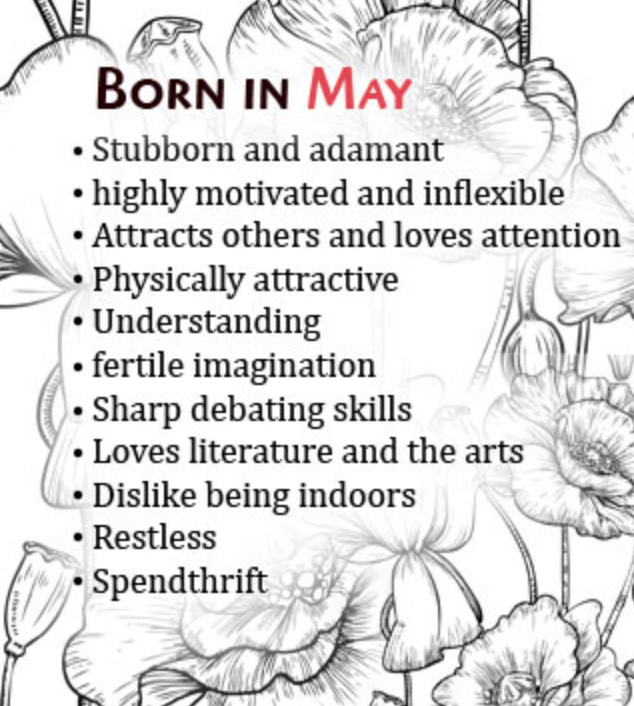table div table+table+table+table+table+table+table+table+table+table+table+table+table+table+table+table+table+table+table+table+table+table+table+table+table+table+table+table+table+table+table+table+table+table+table+table div table{width:100%;padding:0}table div table+table+table+table+table+table+table+table+table+table+table+table+table+table+table+table+table+table+table+table+table+table+table+table+table+table+table+table+table+table+table+table+table+table+table+table div table img{width:96.23%;padding:0;float:none}table div table+table+table+table+table+table+table+table+table+table+table+table+table+table+table+table+table+table+table+table+table+table+table+table+table+table+table+table+table+table+table+table+table+table+table+table div table td{width:100%;padding:0 1.88% 18px}/* styles */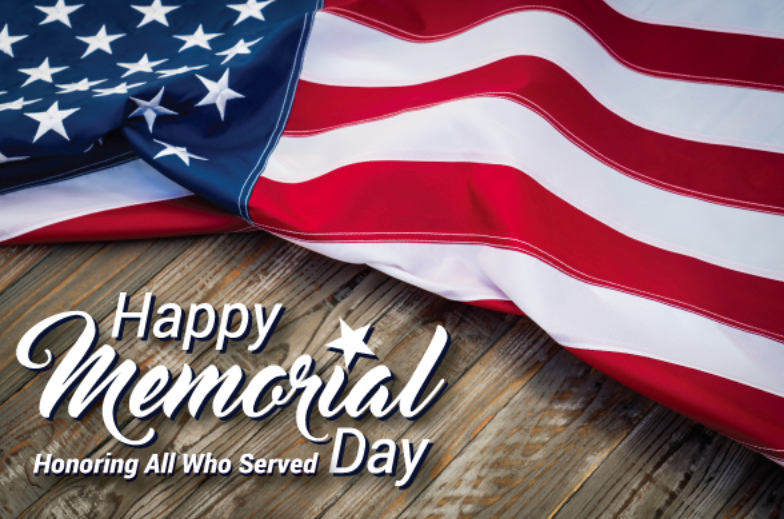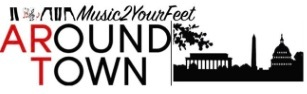/* styles */ Music2YourFeet visits many areas within the community, centers, parks and other events - spreading our love of line dancing. We capture every moment with video and photos. Please check out our photo gallery each month - you may find yourself in them - "Aroundtown"! Special Thank you To front line employees who are helping to keep us safe, we love and appreciate you.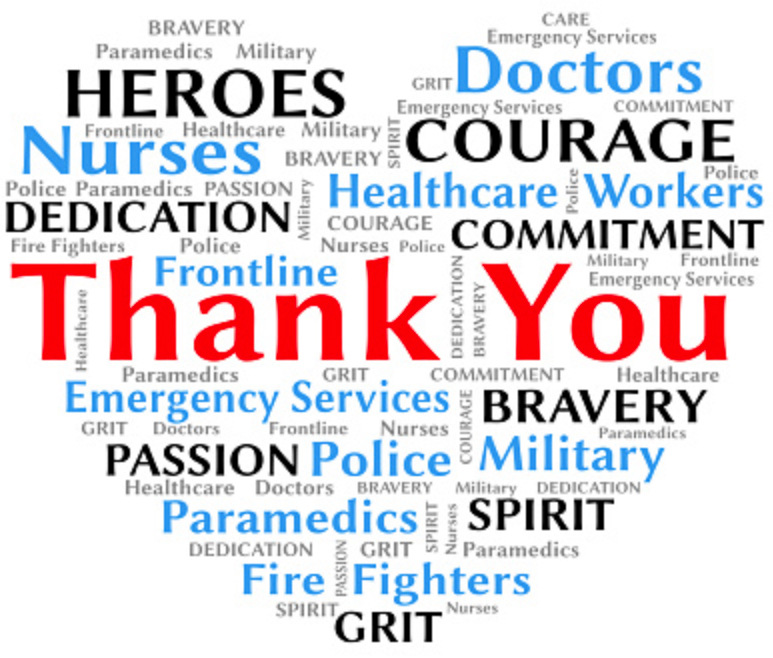table div table+table+table+table+table+table+table+table+table+table+table+table+table+table+table+table+table+table+table+table+table+table+table+table+table+table+table+table+table+table+table+table+table+table+table+table+table+table+table+table+table+table div table{width:100%;padding:0}table div table+table+table+table+table+table+table+table+table+table+table+table+table+table+table+table+table+table+table+table+table+table+table+table+table+table+table+table+table+table+table+table+table+table+table+table+table+table+table+table+table+table div table img{width:96.23%;padding:0;float:none}table div table+table+table+table+table+table+table+table+table+table+table+table+table+table+table+table+table+table+table+table+table+table+table+table+table+table+table+table+table+table+table+table+table+table+table+table+table+table+table+table+table+table div table td{width:100%;padding:0 1.88% 18px}/* styles */table.module-43{width:75.47%;padding:0}table div table+table+table+table+table+table+table+table+table+table+table+table+table+table+table+table+table+table+table+table+table+table+table+table+table+table+table+table+table+table+table+table+table+table+table+table+table+table+table+table+table+table+table+table div table{width:75.47%;float:none;margin-left:auto;margin-right:auto;padding:0}table div table+table+table+table+table+table+table+table+table+table+table+table+table+table+table+table+table+table+table+table+table+table+table+table+table+table+table+table+table+table+table+table+table+table+table+table+table+table+table+table+table+table+table+table div table a{border:0 none;text-decoration:none}table div table+table+table+table+table+table+table+table+table+table+table+table+table+table+table+table+table+table+table+table+table+table+table+table+table+table+table+table+table+table+table+table+table+table+table+table+table+table+table+table+table+table+table+table div table img{width:100%!important;border:0 none;text-decoration:none}table div table+table+table+table+table+table+table+table+table+table+table+table+table+table+table+table+table+table+table+table+table+table+table+table+table+table+table+table+table+table+table+table+table+table+table+table+table+table+table+table+table+table+table+table div table td{width:100%;padding:0}/* styles */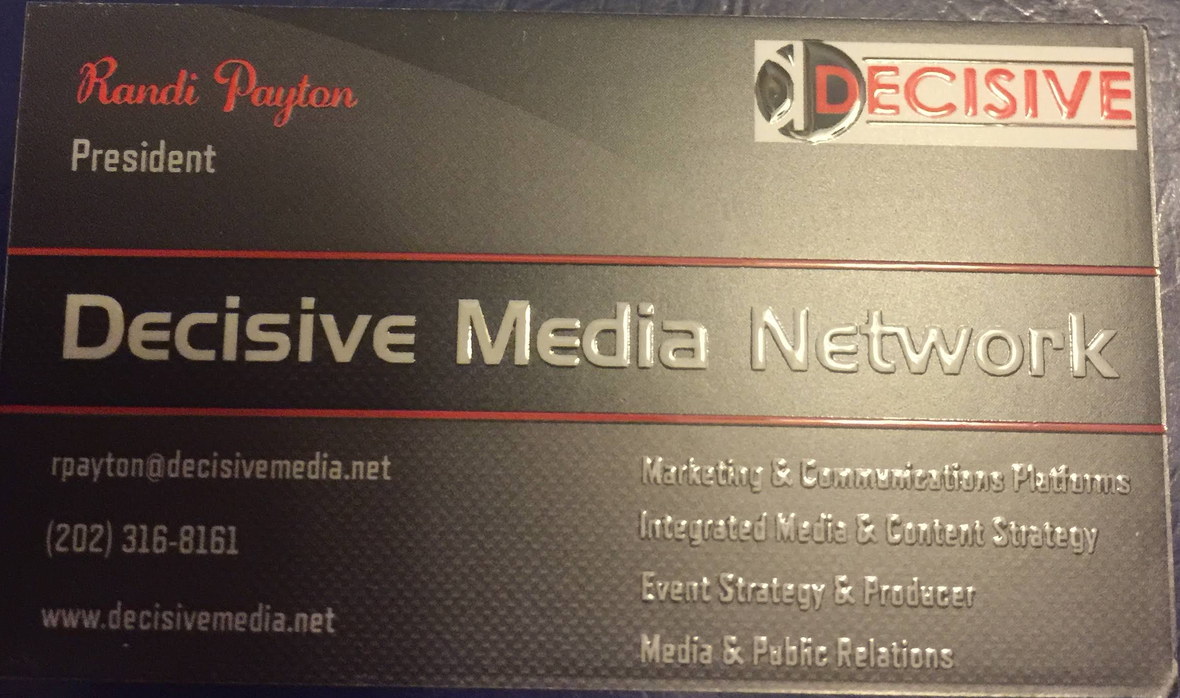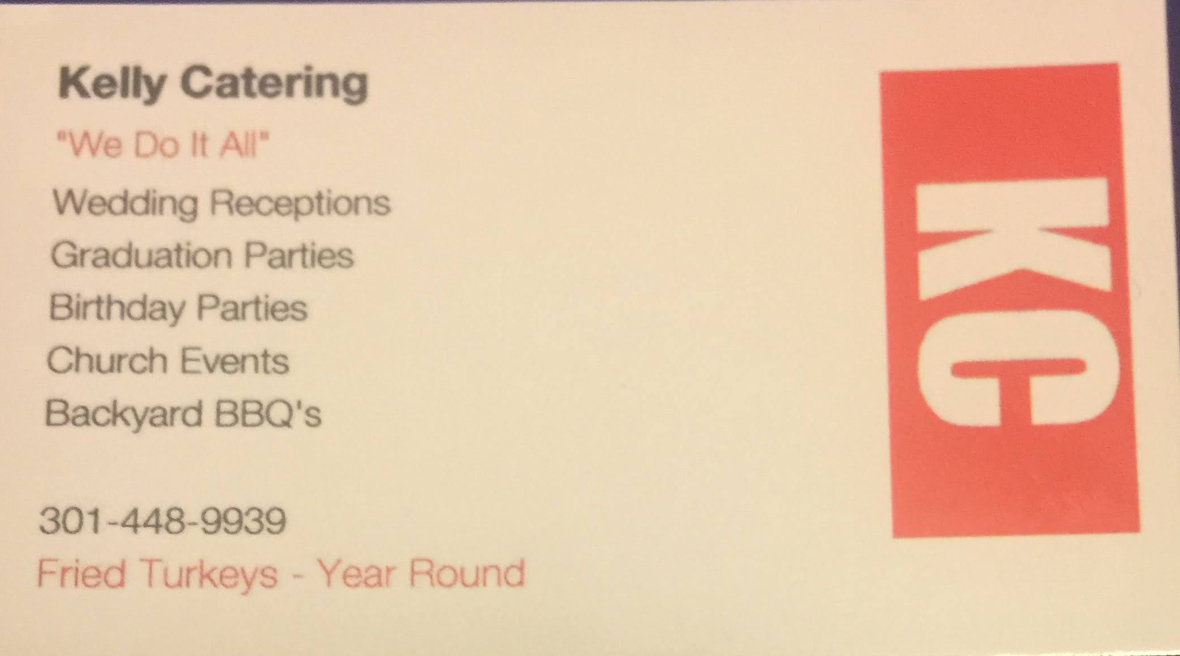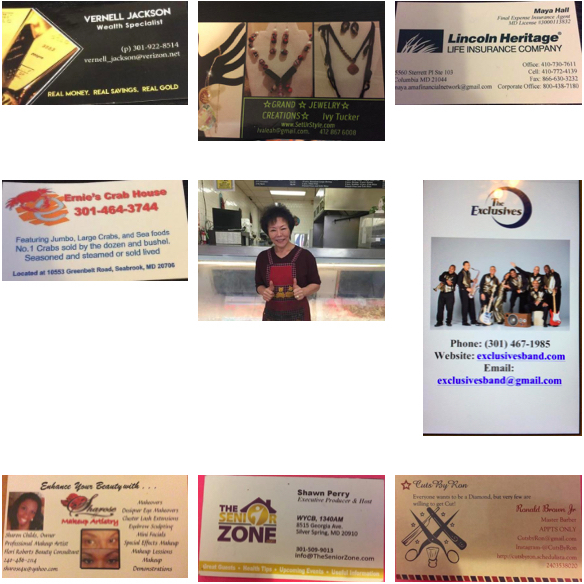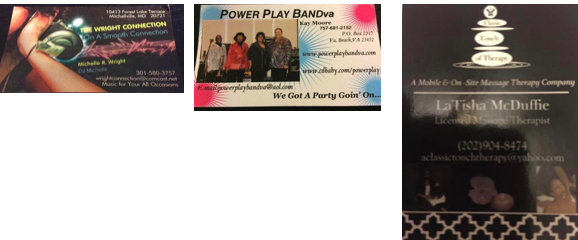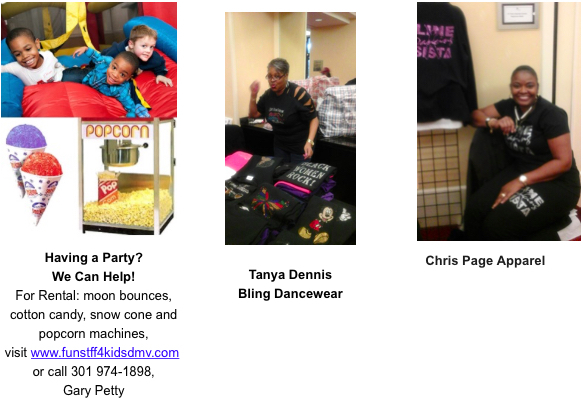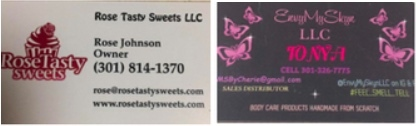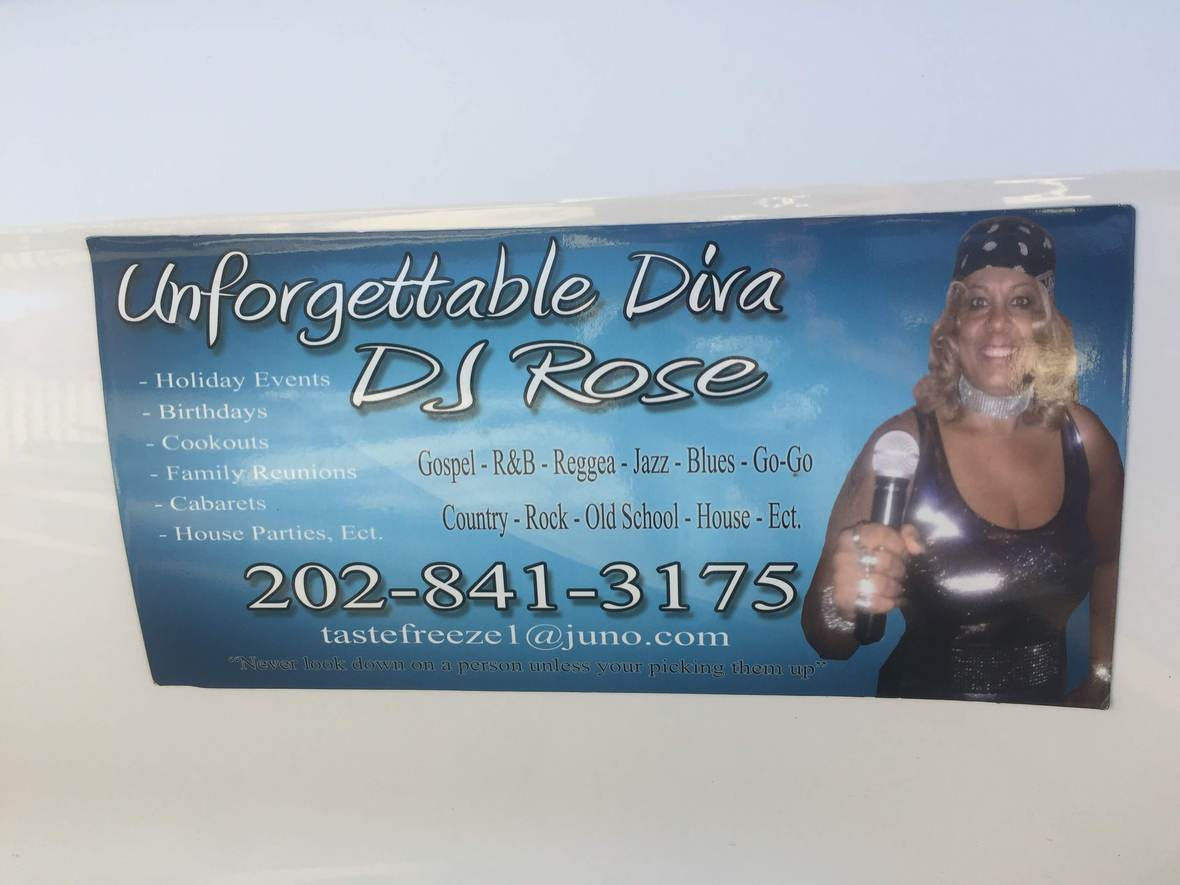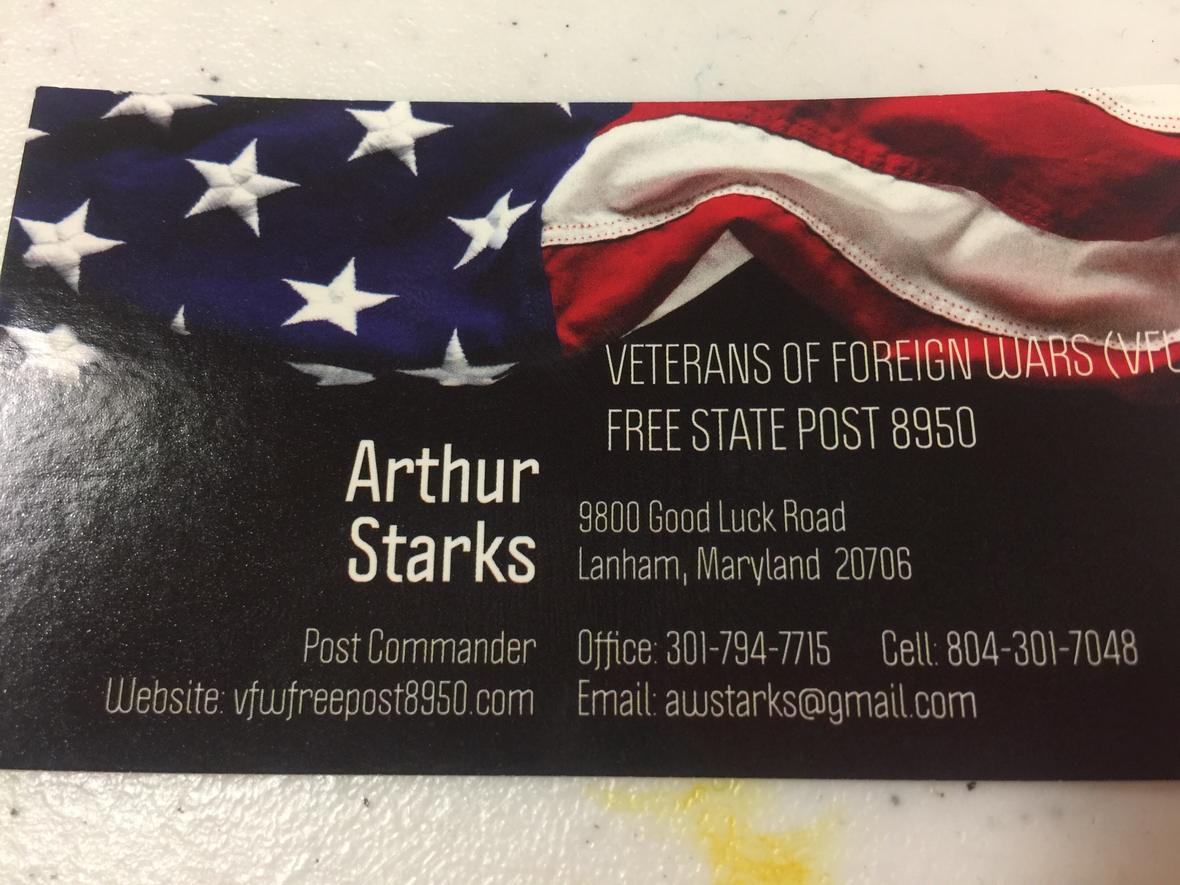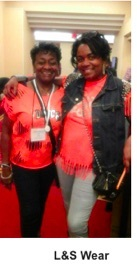# Thank you for your continued support!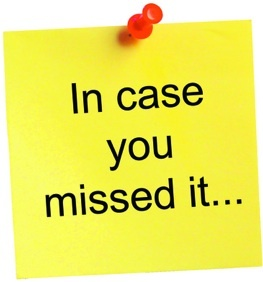Classes and Social Events Cancelled Due to the Coronavirus Outbreak
As positive coronavirus cases have increased in Maryland and Gov. Larry Hogan (R) has declared a state of emergency, which has restricted public gatherings, businesses and government facilities, most public events have been canceled or postponed and public access to government services are increasingly restricted. With that all dance classes and social dance events are cancelled. Please check our website and Facebook page for updates.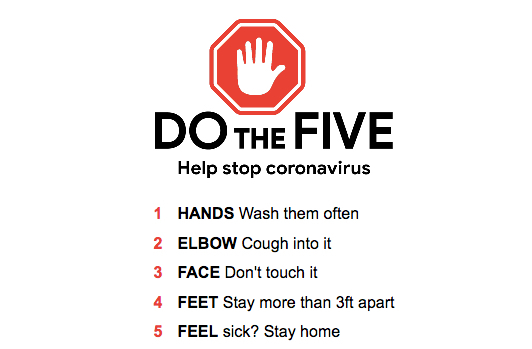table div table+table+table+table+table+table+table+table+table+table+table+table+table+table+table+table+table+table+table+table+table+table+table+table+table+table+table+table+table+table+table+table+table+table+table+table+table+table+table+table+table+table+table+table+table+table+table+table+table+table+table+table+table+table+table+table+table div table{width:100%;padding:0}table div table+table+table+table+table+table+table+table+table+table+table+table+table+table+table+table+table+table+table+table+table+table+table+table+table+table+table+table+table+table+table+table+table+table+table+table+table+table+table+table+table+table+table+table+table+table+table+table+table+table+table+table+table+table+table+table+table div table img{width:96.23%;padding:0;float:none}table div table+table+table+table+table+table+table+table+table+table+table+table+table+table+table+table+table+table+table+table+table+table+table+table+table+table+table+table+table+table+table+table+table+table+table+table+table+table+table+table+table+table+table+table+table+table+table+table+table+table+table+table+table+table+table+table+table div table td{width:100%;padding:0 1.88% 18px}/* styles */## Music2YourFeet Classes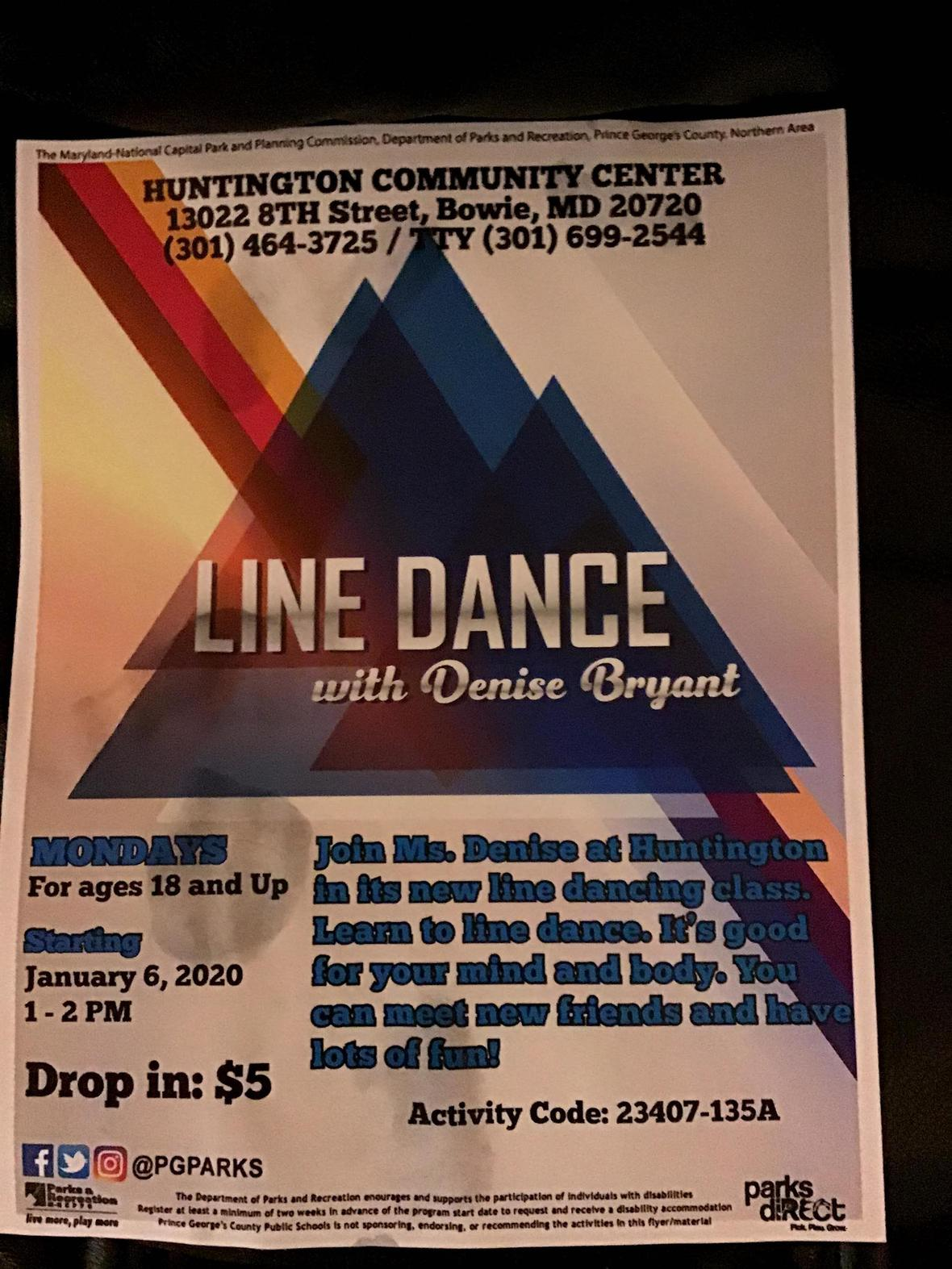Music2yourfeet line dance is at Huntington Community Center/Old Antique Bowie!

Beginners and Seniors, but all are welcome....come out for some fun and exercise, no experience necessary...every Monday at 1:00, see you there.

When: Mondays 1:00-2:00, \$5 drop in
Where: 13022 8th Street, Bowie, Md.

 /* styles */ Monday Lake Arbor Community Center 10100 Lake Arbor Way, Mitchellville, Md. 20721 10:00am-11:30am Calling all Beginners & Seniors, come out for some fun and exercise with Denise Monday Huntington Community Center 13022 8th Street Bowie, MD 1:00pm - 2:00pm \$5 drop in Tuesday Cedar Heights Community Center 1200 Glen Willow Drive Seat Pleasant, MD 9:00am-10:30am \$6, Intermediate Tuesday Glenn Dale Community Center 11901 Glenn Dale Blvd, Glenn Dale, MD 20769 11-12:30pm Beginner \$20 for residents and \$24 for Non-residents. \$5 drop in fee. Beginner Wednesday Good Luck Community Ctr. 6:30-8:00pm 8601 Good Luck Rd, Lanham, Md. 20706 Beginner Friday Ceder Heights Community Center 9am-10:30am 1200 Glen Willow Dr, Capitol Heights, MD 20743 Beginner Friday Senior Day Good Luck Community Center (on-going) 8601 Good Luck Rd Lanham, Md. 11:30am-1:30pm Seniors 60+ FREE, under 60 yrs. Old \$6 Music2YourFeet Private Lessons Available
 table div table+table+table+table+table+table+table+table+table+table+table+table+table+table+table+table+table+table+table+table+table+table+table+table+table+table+table+table+table+table+table+table+table+table+table+table+table+table+table+table+table+table+table+table+table+table+table+table+table+table+table+table+table+table+table+table+table+table+table+table+table+table div table{width:100%;padding:0}table div table+table+table+table+table+table+table+table+table+table+table+table+table+table+table+table+table+table+table+table+table+table+table+table+table+table+table+table+table+table+table+table+table+table+table+table+table+table+table+table+table+table+table+table+table+table+table+table+table+table+table+table+table+table+table+table+table+table+table+table+table+table div table img{width:96.23%;padding:0;float:none}table div table+table+table+table+table+table+table+table+table+table+table+table+table+table+table+table+table+table+table+table+table+table+table+table+table+table+table+table+table+table+table+table+table+table+table+table+table+table+table+table+table+table+table+table+table+table+table+table+table+table+table+table+table+table+table+table+table+table+table+table+table+table div table td{width:100%;padding:0 1.88% 18px}/* styles */## DMV Monthly Dance Socials

 /* styles */ First Sunday of Each Month Sunday Line Dance Social - at Cedar Heights Community Center, 1200 Glen Willow Drive, Seat Pleasant, Md. 3pm - 7pm \$6 for Res & Non-Res. First Friday Line Dance Socials with April Love Jones Southern Regional Technology and Recreation Complex, 6pm-9:30pm includes light refreshments, \$5 resident \$6 non resident First and Third Friday Delight featuring DJ Zone @ VFW Post 7916 (upstairs), 204 Mill Street Occoquan, Va 22125. Time: 7:30 PM to 11:30 PM. Cost: \$10. Open dancing, Cash Bar, Bring your own Food or purchase from the VFW. Follow DJ Zone on Facebook under Vincent Price and join my DJ Zone Status Group for other events and dates. First Saturday of Each Month - Toast it Up Productions - Shut Up & Dance, 1:00-5:00pm, Elk's Lodge, 7350 Temple Hills, Md. BYOF, \$7.00 Third Saturday of Each Month S.O.S. (Seniors On Step) line dance social; 6:00-10:00pm; Cost: Free; BYOFood & beverage (no alcohol permitted) Kingstowne Community Center for Active Adults, 6488 Landsdowne Centre Drive, Alexandria, VA 22315. Every Second Friday, \$5 6p-9p. Learn the latest line dances with Peytrienne! All are welcome, water provided, Soul Line & Hand dance Party, White Oak Community Center, 1700 April Lane Silver Spring, Md. 20904 Every Sunday Elks Lodge of Camp Springs 7350 Temple Hills Rd Temple Hills Md, Hand Dance, Bop, Line Dance & more 6p-10p Fourth Friday of the Month - Style & Rhythm, 4th Friday social ,\$5, Ridgley's Run Community Ctr. 8400 Mission Road, Jessup, Md.
 table div table+table+table+table+table+table+table+table+table+table+table+table+table+table+table+table+table+table+table+table+table+table+table+table+table+table+table+table+table+table+table+table+table+table+table+table+table+table+table+table+table+table+table+table+table+table+table+table+table+table+table+table+table+table+table+table+table+table+table+table+table+table+table+table+table div table{width:100%;padding:0}table div table+table+table+table+table+table+table+table+table+table+table+table+table+table+table+table+table+table+table+table+table+table+table+table+table+table+table+table+table+table+table+table+table+table+table+table+table+table+table+table+table+table+table+table+table+table+table+table+table+table+table+table+table+table+table+table+table+table+table+table+table+table+table+table+table div table img{width:96.23%;padding:0;float:none}table div table+table+table+table+table+table+table+table+table+table+table+table+table+table+table+table+table+table+table+table+table+table+table+table+table+table+table+table+table+table+table+table+table+table+table+table+table+table+table+table+table+table+table+table+table+table+table+table+table+table+table+table+table+table+table+table+table+table+table+table+table+table+table+table+table div table td{width:100%;padding:0 1.88% 18px}/* styles *//* styles */ Denise's "Music2YourFeet" Line Dancing provides you with the opportunity to exercise socialize and have fun - all in one setting. Break the monotony of a long day at work, (evening classes); get mid-day relief to an otherwise stressful day (lunch time classes); Relax after a long work week (weekend classes); or one-on-one personal service (private classes, no matter the day, it will be music2yourfeet Submit your events. Donations not required but appreciated.
 table div table+table+table+table+table+table+table+table+table+table+table+table+table+table+table+table+table+table+table+table+table+table+table+table+table+table+table+table+table+table+table+table+table+table+table+table+table+table+table+table+table+table+table+table+table+table+table+table+table+table+table+table+table+table+table+table+table+table+table+table+table+table+table+table+table+table+table+table div table{width:100%;padding:0}table div table+table+table+table+table+table+table+table+table+table+table+table+table+table+table+table+table+table+table+table+table+table+table+table+table+table+table+table+table+table+table+table+table+table+table+table+table+table+table+table+table+table+table+table+table+table+table+table+table+table+table+table+table+table+table+table+table+table+table+table+table+table+table+table+table+table+table+table div table img{width:96.23%;padding:0;float:none}table div table+table+table+table+table+table+table+table+table+table+table+table+table+table+table+table+table+table+table+table+table+table+table+table+table+table+table+table+table+table+table+table+table+table+table+table+table+table+table+table+table+table+table+table+table+table+table+table+table+table+table+table+table+table+table+table+table+table+table+table+table+table+table+table+table+table+table+table div table td{width:100%;padding:0 1.88% 18px}/* styles */## More Line Dance Classes

 /* styles */ Garland Christopher Tuesday only class 11:00a-12:00pm Palmer Park Community Center 7720 Barlowe Rd, Landover, MD 20785 (301) 773-5665
 table div table+table+table+table+table+table+table+table+table+table+table+table+table+table+table+table+table+table+table+table+table+table+table+table+table+table+table+table+table+table+table+table+table+table+table+table+table+table+table+table+table+table+table+table+table+table+table+table+table+table+table+table+table+table+table+table+table+table+table+table+table+table+table+table+table+table+table+table+table+table+table div table{width:100%;padding:0}table div table+table+table+table+table+table+table+table+table+table+table+table+table+table+table+table+table+table+table+table+table+table+table+table+table+table+table+table+table+table+table+table+table+table+table+table+table+table+table+table+table+table+table+table+table+table+table+table+table+table+table+table+table+table+table+table+table+table+table+table+table+table+table+table+table+table+table+table+table+table+table div table img{width:96.23%;padding:0;float:none}table div table+table+table+table+table+table+table+table+table+table+table+table+table+table+table+table+table+table+table+table+table+table+table+table+table+table+table+table+table+table+table+table+table+table+table+table+table+table+table+table+table+table+table+table+table+table+table+table+table+table+table+table+table+table+table+table+table+table+table+table+table+table+table+table+table+table+table+table+table+table+table div table td{width:100%;padding:0 1.88% 18px}/* styles */## Upcoming Events

 /* styles */ Classes and Social Events Cancelled Due to the Coronavirus Outbreak As positive coronavirus cases have increased in Maryland and Gov. Larry Hogan (R) has declared a state of emergency, which has restricted public gatherings, businesses and government facilities, most public events have been canceled or postponed and public access to government services are increasingly restricted. With that all dance classes and social dance events are cancelled. Please check our website and Facebook page for updates.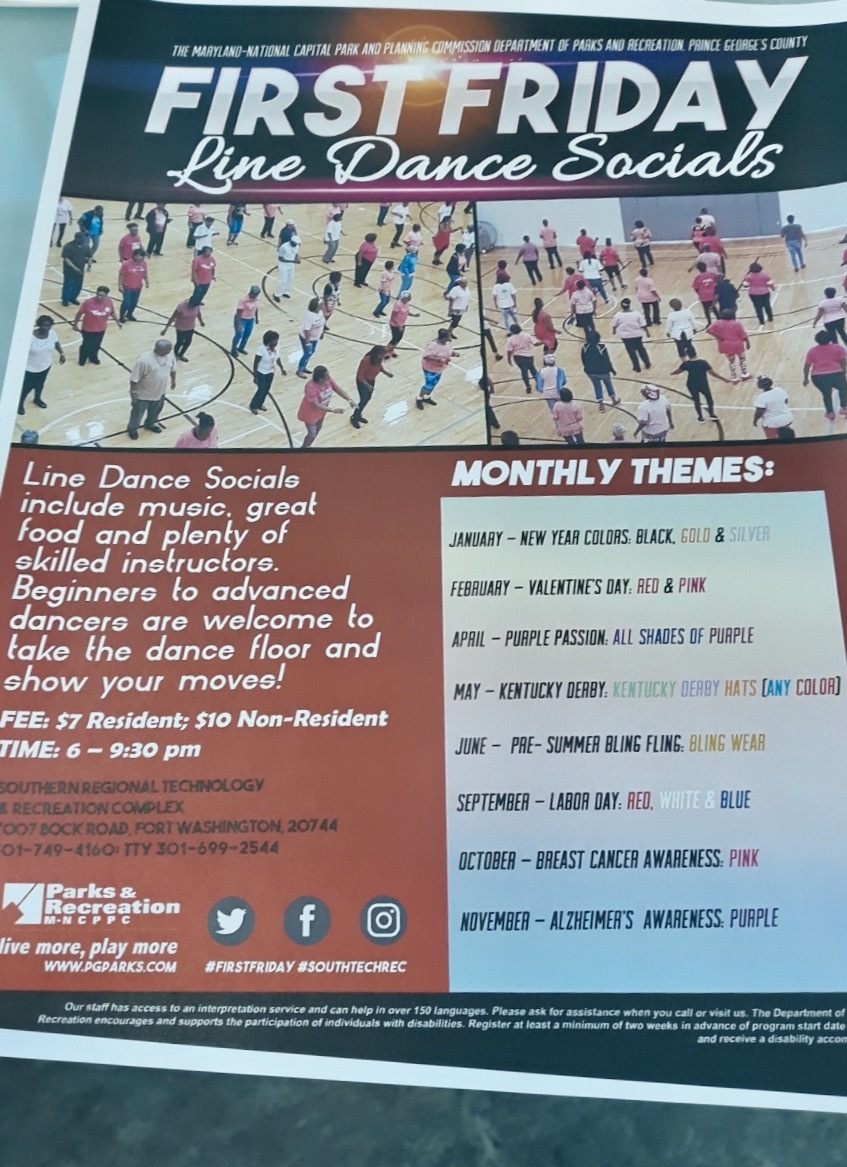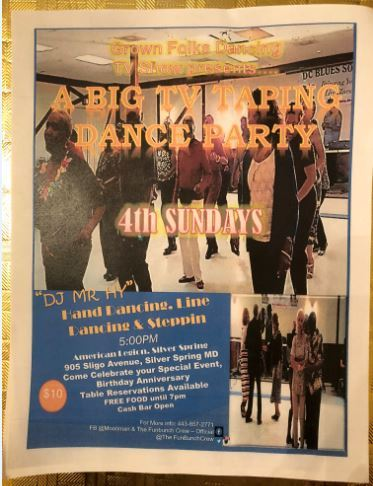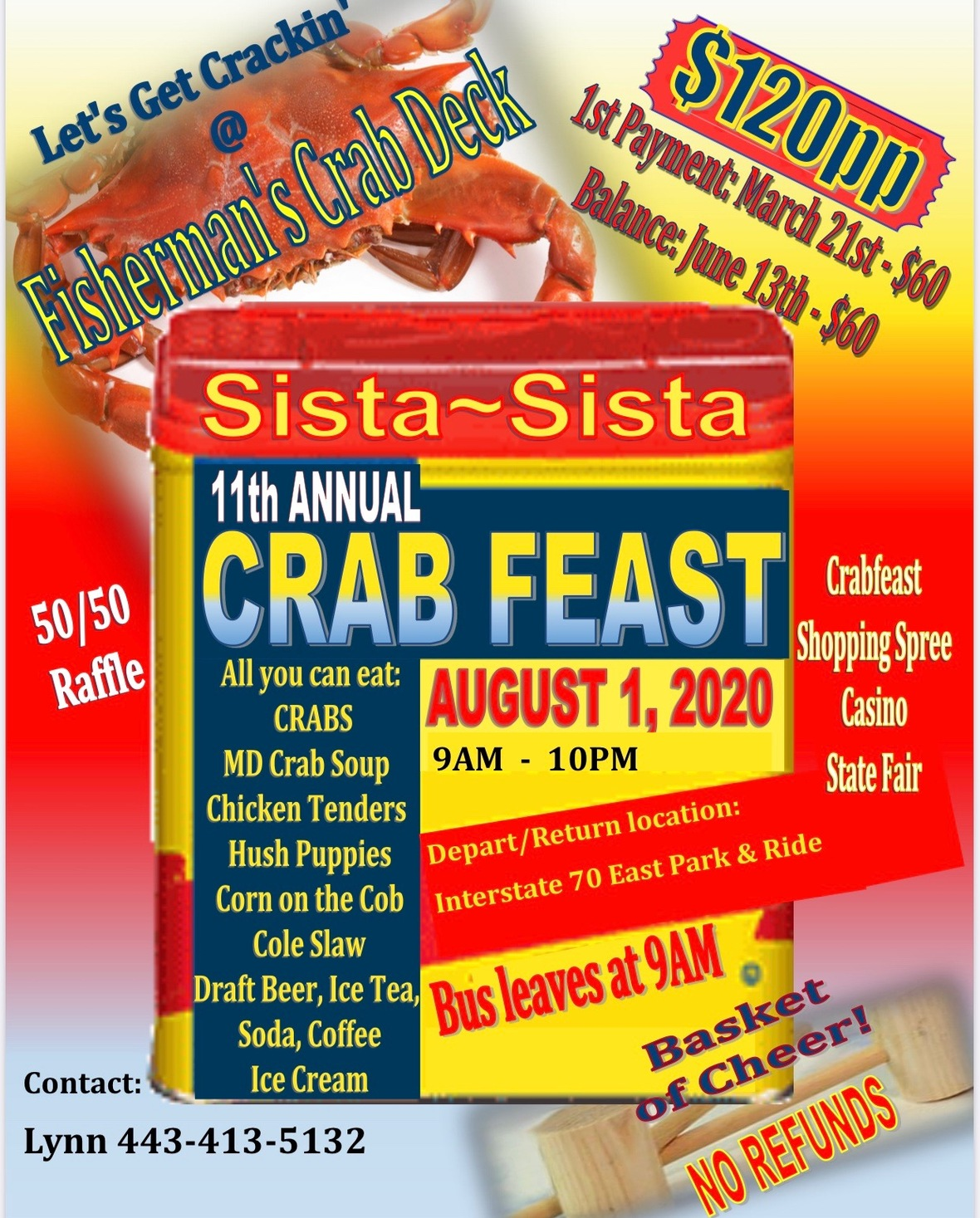table div table+table+table+table+table+table+table+table+table+table+table+table+table+table+table+table+table+table+table+table+table+table+table+table+table+table+table+table+table+table+table+table+table+table+table+table+table+table+table+table+table+table+table+table+table+table+table+table+table+table+table+table+table+table+table+table+table+table+table+table+table+table+table+table+table+table+table+table+table+table+table+table+table+table+table+table+table+table div table{width:100%;padding:0}table div table+table+table+table+table+table+table+table+table+table+table+table+table+table+table+table+table+table+table+table+table+table+table+table+table+table+table+table+table+table+table+table+table+table+table+table+table+table+table+table+table+table+table+table+table+table+table+table+table+table+table+table+table+table+table+table+table+table+table+table+table+table+table+table+table+table+table+table+table+table+table+table+table+table+table+table+table+table div table img{width:96.23%;padding:0;float:none}table div table+table+table+table+table+table+table+table+table+table+table+table+table+table+table+table+table+table+table+table+table+table+table+table+table+table+table+table+table+table+table+table+table+table+table+table+table+table+table+table+table+table+table+table+table+table+table+table+table+table+table+table+table+table+table+table+table+table+table+table+table+table+table+table+table+table+table+table+table+table+table+table+table+table+table+table+table+table div table td{width:100%;padding:0 1.88% 18px}/* styles */